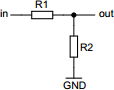# Resistive voltage divider

## Calculates properties of resistive voltage divider circuit

 Example 1: Must calculate the input voltage when output voltage is 500 millivolts using 120 kilo-ohm source and 10 kilo-ohm sink resistors View example Example 2: Must calculate the source resistor to have 1 volt output at 100 volt input using 2.2 kilo-ohm sink resistor View example
 Input voltage volts Output voltage volts Source resistor (R1) ohms Sink resistor (R2) ohmsYou may use one of the following SI prefix after a value: p=pico, n=nano, u=micro, m=milli, k=kilo, M=mega, G=giga

Fill in all values except the one you wish to calculate
• Input voltage - Input voltage of the source
• Output voltage - Output voltage of the divider
• Source resistor - Resistor connected between input and output (R1)
• Sink resistor - Resistor connected between output and ground (R2)